# PySpark Tutorial: Learn Apache Spark Using Python

### A discussion of the open source Apache Spark platform, and a tutorial on to use it with Python for big data processes. Read on for more!

· Big Data Zone · Tutorial
Save
31.65K Views

In a world where data is being generated at such an alarming rate, the correct analysis of that data at the correct time is very useful. One of the most amazing frameworks to handle big data in real-time and perform analyses is Apache Spark, And if we talk about the programming languages being used nowadays for handling complex data analysis and data munging tasks, I'm sure Python will top this chart. So in this PySpark tutorial, I'll discuss the following topics:

• What is PySpark?
• PySpark in the Industry
• Why Go for Python?
• Spark RDDs
• Machine Learning with PySpark

## PySpark Tutorial: What Is PySpark?

Apache Spark is a fast cluster computing framework which is used for processing, querying and analyzing big data. Being based on in-memory computation, it has an advantage over several other big data frameworks.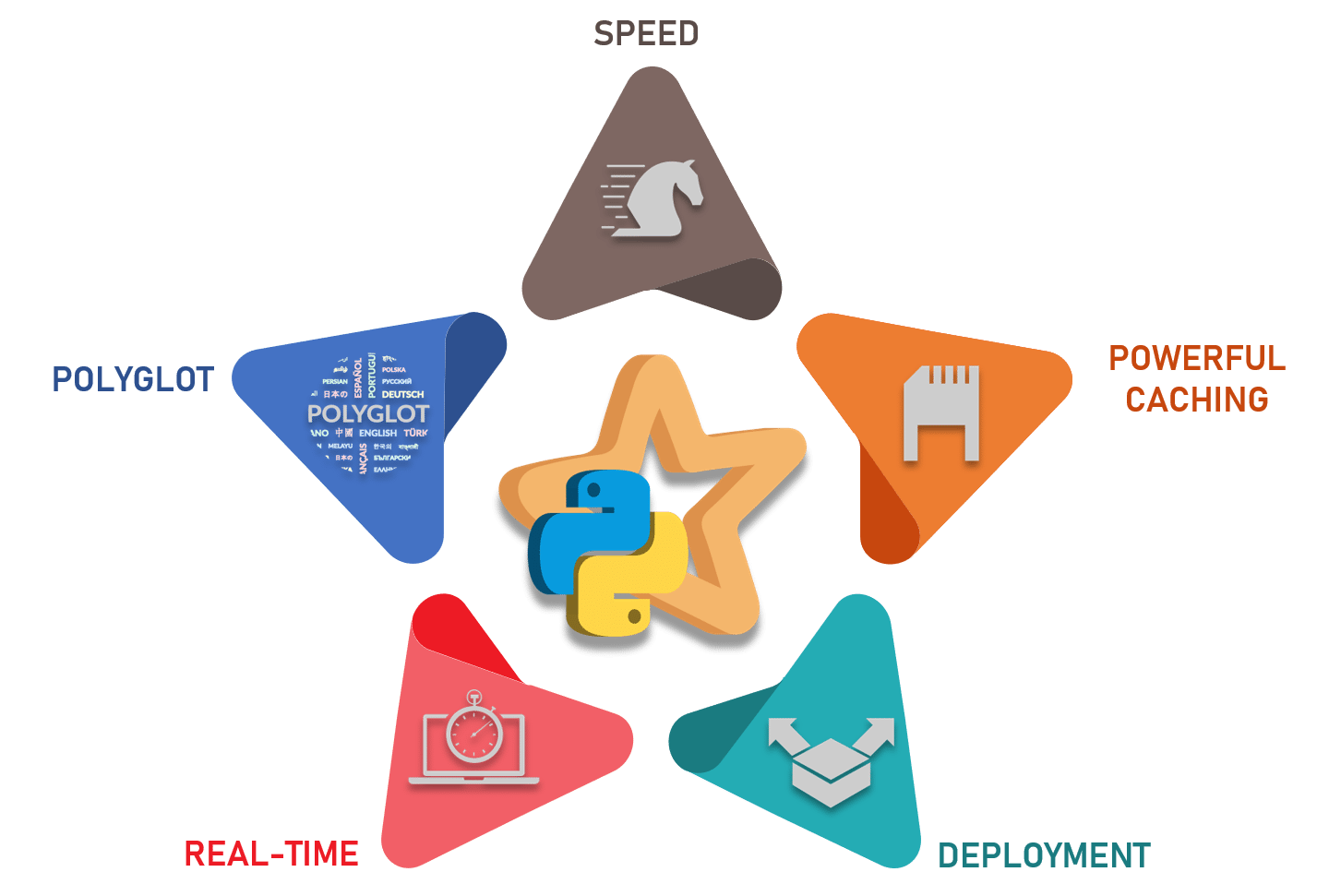Originally written in the Scala programming language, the open source community has developed an amazing tool to support Python for Apache Spark. PySpark helps data scientists interface with RDDs in Apache Spark and Python through its library Py4j. There are many features that make PySpark a better framework than others:

• Speed: It is 100x faster than traditional large-scale data processing frameworks.
• Powerful Caching: Simple programming layer provides powerful caching and disk persistence capabilities.
• Deployment: Can be deployed through Mesos, Hadoop via Yarn, or Spark's own cluster manager.
• Real Time: Real-time computation and low latency because of in-memory computation.
• Polyglot: Supports programming in Scala, Java, Python, and R.

Let's move ahead with our PySpark Tutorial Blog and see where is Spark used in the industry.

## PySpark in the Industry

Let's move ahead with our PySpark tutorial and see where Spark is used in the industry.

Every industry revolves around big data and where there's big data, there's analysis involved. So let's have a look at the various industries where Apache Spark is used.Media is one of the biggest industries growing towards online streaming. Netflix uses Apache Spark for real-time stream processing to provide personalized online recommendations to its customers. It processes 450 billion events per day which flow to server-side applications.Finance is another sector where Apache Spark's real-time processing plays an important role. Banks are using Spark to access and analyze social media profiles to gain insights which can help them make the right business decisions for credit risk assessment, targeted ads, and customer segmentation. Customer churn is also reduced using Spark. Fraud detection is one of the most widely used areas of machine learning where Spark is involved.Healthcare providers are using Apache Spark to analyze patient records along with past clinical data to identify which patients are likely to face health issues after being discharged from the clinic. Apache Spark is used in genomic sequencing to reduce the time needed to process genome data.Retail and e-commerce is an industry where one can't imagine it running without the use of analysis and targeted advertising. One of the largest e-commerce platform today, Alibabaruns some of the largest Spark jobs in the world in order to analyze petabytes of data. Alibaba performs feature extraction in image data. eBay uses Apache Spark to provide targeted offers, enhance customer experience and optimize overall performance.Travel industries also use Apache Spark. TripAdvisor, a leading travel website that helps users plan a perfect trip, is using Apache Spark to speed up its personalized customerrecommendations. TripAdvisor uses Apache Spark to provide advice to millions of travelers by comparing hundreds of websites to find the best hotel prices for its customers.

An important aspect of this PySpark tutorial is to understand why we need to use Python. Why not Java, Scala or R?

Easy to Learn: For programmers, Python is comparatively easier to learn because of its syntax and standard libraries. Moreover, it's a dynamically typed language, which means RDDs can hold objects of multiple types.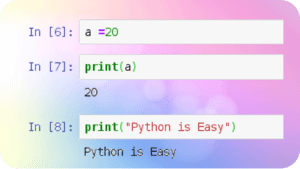A vast set of libraries: Scala does not have sufficient data science tools and libraries like Python for machine learning and natural language processing. Moreover, Scala lacks good visualization and local data transformations.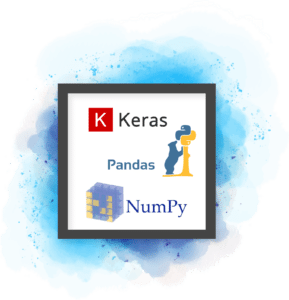Huge Community Support: Python has a global community with millions of developers that interact online and offline in thousands of virtual and physical locations.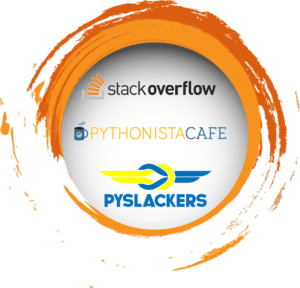One of the most important topics in this PySpark tutorial is the use of RDDs. Let's understand what RDDs are.

## Spark RDDs

When it comes to iterative distributed computing, i.e. processing data over multiple jobs in computations, we need to reuse or share data among multiple jobs. Earlier frameworks like Hadoop had problems while dealing with multiple operations/jobs like:

• Storing data in intermediate storage such as HDFS.
• Multiple I/O jobs make the computations slow.
• Replications and serializations which in turn makes the process even slower.

RDDs try to solve all the problems by enabling fault-tolerant distributed in-memory computations. RDD is short for Resilient Distributed Datasets. RDD is a distributed memory abstraction which lets programmers perform in-memory computations on large clusters in a fault-tolerant manner. They are the read-only collection of objects partitioned across a set of machines that can be rebuilt if a partition is lost. There are several operations performed on RDDs:

• Transformations: Transformations create a new dataset from an existing one. Lazy Evaluation.
• Actions: Spark forces the calculations for execution only when actions are invoked on the RDDs.

Let's understand a few Transformations, Actions, and Functions.

### Reading a File and Displaying Top n Elements:

``````rdd = sc.textFile("file:///home/edureka/Desktop/Sample")
rdd.take(n)``````
``````[u'Deforestation is arising as the main environmental and social issue which has now taken the form of more than a powerful demon. ',
u'We must know about the causes, effects and ways to solve the problems arisen because of the deforestation. ',
u'We have provided many paragraphs, long and short essay on deforestation in order to help your kids and children to get aware about the problem as well as get participated in the essay writing competition in the school or outside the school. ',
u'You can select any deforestation essay given below according to the class standard. ',
u'Deforestation is arising as the major global problem to the society and environment.']``````

### Converting to Lowercase and Splitting:(Lower and Split)

``````def Func(lines):
lines = lines.lower()
lines = lines.split()
return lines
rdd1 = rdd.map(Func)

rdd1.take(5)``````
``````[[u'deforestation',
u'is',
u'arising',
u'as',
u'the',
u'main',
u'environmental',
u'and',
u'social',
u'issue',
u'which',
u'has',
u'now',
u'taken',
.....
.
.
.
]``````

### Removing StopWords:(Filter)

``````stop_words = ['a','all','the','as','is','am','an','and','be','been','from','had','I','I'd','why','with']
rdd2 = rdd1.filter(lambda z: z not in stop_words)
rdd2.take(10)``````
``````[u'deforestation',
u'arising',
u'main',
u'environmental',
u'social',
u'issue',
u'which',
u'has',
u'now',
u'taken']``````

### Sum of Numbers from 1 to 500: (Reduce)

``````sum_rdd = sc.parallelize(range(1,500))
sum_rdd.reduce(lambda x,y: x+y)``````
``124750``

## Machine Learning With PySpark

Continuing our PySpark tutorial, let's analyze some basketball data and make some predictions. So, here we are going to use data of all the players in NBA since 1980 [year of introduction of 3 Pointers].``````df = spark.read.option('header','true')\
.option('inferSchema','true')
``print(df.columns)``
``['_c0', 'player', 'pos', 'age', 'team_id', 'g', 'gs', 'mp', 'fg', 'fga', 'fg_pct', 'fg3', 'fg3a', 'fg3_pct', 'fg2', 'fg2a', 'fg2_pct', 'efg_pct', 'ft', 'fta', 'ft_pct', 'orb', 'drb', 'trb', 'ast', 'stl', 'blk', 'tov', 'pf', 'pts', 'yr']``

### Sorting Players (`OrderBy`) and `toPandas`:

Here we are sorting players based on points scored in a season.

``df.orderBy('pts',ascending = False).limit(10).toPandas()[['yr','player','age','pts','fg3']]``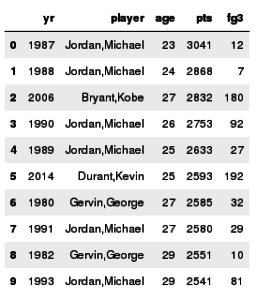### Using DSL and matplotlib:

Here we are analyzing the average number of 3 point attempts for each season in a time limit of 36 min [an interval corresponding to an approximate full NBA game with adequate rest]. We compute this metric using the number of 3-point field goal attempts (fg3a) and minutes played (mp) and then plot the result using `matlplotlib`.

``````from pyspark.sql.functions import col
fga_py = df.groupBy('yr')\
.agg({'mp' : 'sum', 'fg3a' : 'sum'})
.select(col('yr'), (36*col('sum(fg3a)')/col('sum(mp)')).alias('fg3a_p36m'))\
.orderBy('yr')

from matplotlib import pyplot as plt
import seaborn as sns
plt.style.use('fivethirtyeight')

_df = fga_py.toPandas()
plt.plot(_df.yr,_df.fg3a_p36m, color = '#CD5C5C')
plt.xlabel('Year')
_=plt.title('Player average 3-point attempts (per 36 minutes)')
plt.annotate('3 pointer introduced', xy=(1980, .5), xytext=(1981, 1.1), fontsize = 9,
arrowprops=dict(facecolor='grey', shrink=0, linewidth = 2))
plt.annotate('NBA moved in 3-point line', xy=(1996, 2.4), xytext=(1991.5, 2.7), fontsize = 9,
arrowprops=dict(facecolor='grey', shrink=0, linewidth = 2))
plt.annotate('NBA moved back\n3-point line', xy=(1998, 2.), xytext=(1998.5, 2.4), fontsize = 9, arrowprops=dict(facecolor='grey', shrink=0, linewidth = 2))``````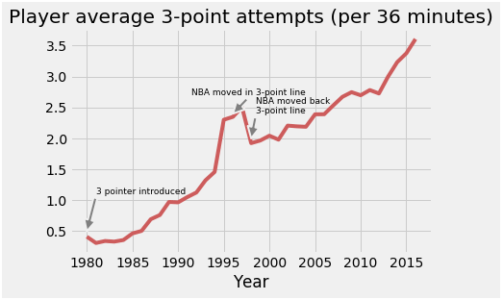### Linear Regression and VectorAssembler:

We can fit a linear regression model to this curve to model the number of shot attempts for the next 5 years. We have to transform our data using the `VectorAssembler` function to a single column. This is a requirement for the linear regression API in MLlib.

``````from pyspark.ml.feature import VectorAssembler
t = VectorAssembler(inputCols=['yr'], outputCol = 'features')
training = t.transform(fga_py)\
.withColumn('yr',fga_py.yr)\
.withColumn('label',fga_py.fg3a_p36m)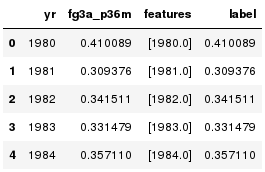We then build our linear regression model object using our transformed data.

``````from pyspark.ml.regression import LinearRegression
lr = LinearRegression(maxIter=10)
model = lr.fit(training)``````

### Applying Trained Model to Dataset:

We apply our trained model object model to our original training set along with 5 years of future data:

``````from pyspark.sql.types import Row

# apply model for the 1979-80 season thru 2020-21 season
training_yrs = training.select('yr').rdd.map(lambda x: x).collect()
training_y = training.select('fg3a_p36m').rdd.map(lambda x: x).collect()
prediction_yrs = [2017, 2018, 2019, 2020, 2021]
all_yrs = training_yrs + prediction_yrs

# built testing DataFrame
test_rdd = sc.parallelize(all_yrs)
row = Row('yr')&amp;amp;lt
all_years_features = t.transform(test_rdd.map(row).toDF())

# apply linear regression model
df_results = model.transform(all_years_features).toPandas()``````

### Plotting the Final Prediction:

We can then plot our results and save the graph in a specified location.

``````plt.plot(df_results.yr,df_results.prediction, linewidth = 2, linestyle = '--',color = '#224df7', label = 'L2 Fit')
plt.plot(training_yrs, training_y, color = '#f08080', label = None)
plt.xlabel('Year')
plt.ylabel('Number of attempts')
plt.legend(loc = 4)
_=plt.title('Player average 3-point attempts (per 36 minutes)')
plt.tight_layout()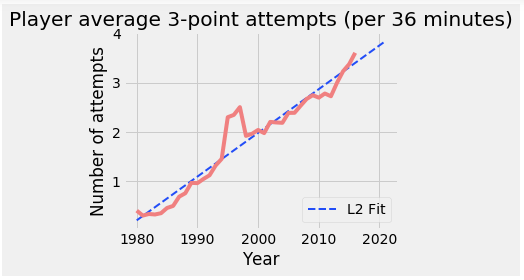And, with this graph, we come to the end of this PySpark tutorial.

So this is it, guys!

I hope you guys got an idea of what PySpark is, why Python is best suited for Spark, the RDDs and a glimpse of machine learning with Pyspark. Congratulations, you are no longer a newbie to PySpark.

Topics:
big data, pyspark, python, apache spark

Published at DZone with permission of Kislay Keshari, DZone MVB.

Opinions expressed by DZone contributors are their own.